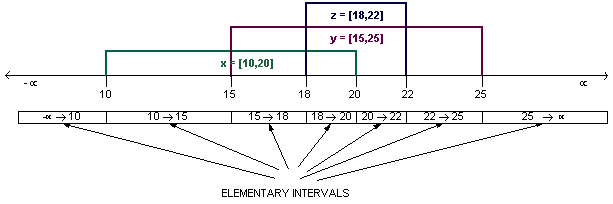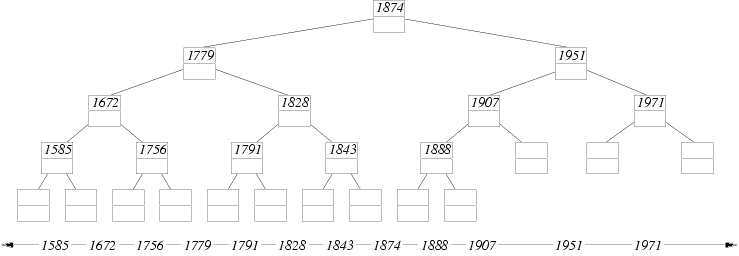CSC 378: Data Structures and Algorithm Analysis

INTERVAL TREES

# Elementary Intervals

An interval is a pair of integers [a,b] such that a < b.

The endpoints a and b of each interval [a,b] divide the integer line into partitions called elementary intervals.

The interval x=[10, 20] has been added to the integer line. Notice that one interval cuts the line at two points:See what happens as we add new intervals. Notice how many new elementary intervals we are creating.Given n intervals [ai, bi], for i = 1 ... n, exactly how many elementary intervals are there, assuming that no intervals [ai,bi] share endpoints?

We get 2n + 1 sub-intervals when there are n intervals on the integer line that do not share endpoints.

Every interval can be expressed as an aggregate of the sub-intervals that it spans:

 Interval spans Sub-Intervals x [10,20] [10,15], [15,18], [18-20] y [15,25] [15,18], [18,20], [20-22], [22,25] z [18, 22] [18,20], [20,22]

# Interval Trees

How would we store these intervals in a data structure?

We would store them in an interval tree. This is an augmentation of the BST data structure.

An interval tree has a leaf node for every elementary interval. On top of these leaves is built a complete binary tree. Each internal node of the tree stores, as its key, the integer that separates the elementary intervals in its left and right subrees. The leaf nodes do not store any keys, and represent the elementary intervals. In the interval tree below, the key is shown in the top of each node.Note that each leaf corresponds to exactly one elementary intervals.

## Insertion of Intervals

How would we store the actual (non-elementary) intervals in this tree?

Each node of the tree (both internal nodes and leaf nodes) can store a set of intervals. In the tree above, this set is shown in the bottom of each node.

We could store these sets as linked lists. Each leaf (which corresponds to an elementary interval) would contain a pointer to a list of non-elementary intervals that span the leaf's elementary interval. For example:What's wrong with this picture?!

It uses up too much space: If each interval were very long, it could potentially be stored in every leaf. Since there are n leaves, we are looking at O(n2) storage!

So how do we improve the storage overhead?

An internal node is said to ``span'' the union of the elementary intervals in its subtree. For example, node 18 spans the interval from 15 to 20: In other words: span(18) = [15,20].

Suppose that, rather than store an interval in each individual leaf, we will store it in the internal nodes. Here is the rule:

An interval [a,b] is stored in a node x if and only if

1)
span(x) is completely contained within [a,b] and
2)
span(parent(x)) is not completely contained in [a,b].

Let's incorporate this rule into our tree:Each interval can be stored at most twice at each level. (Can you prove this?)

This gives us O(n log n) storage - actually 2 n log n, which has a very low coefficient.

MUCH BETTER!

### A More Complex Example

Suppose we have a set of composers and their lifespans; each lifespan is an interval of years between the composer's birth and death. The following table gives an example.

 Interval Composer Birth Death A Stravinsky 1888 1971 B Schoenberg 1874 1951 C Grieg 1843 1907 D Schubert 1779 1828 E Mozart 1756 1791 F Schuetz 1585 1672

The intervals are shown on the integer line. Each interval is labelled with a letter corresponding to one of the composers in the table:Notice that the endpoints a and b of each interval [a,b] divide the integer line into elementary intervals. The elementary intervals for the composers are:

[-infty,1585], [1585,1672], [1672,1756], [1756,1779], ..., [1907,1951], [1951,1971], [1971,+infty].

Here is the Interval Tree for our composers:Notice that node 1828 spans the four elementary intervals between 1779 and 1874. In this case, we say span(1828) = [1779,1874]. A leaf node spans only one elementary interval.

And here's the tree with the intervals stored in each node according to the rule above:For example, the interval C = [1843,1907] corresponds to Grieg's lifetime. This interval is stored in node 1888 because

1)
span(1888) = [1874,1907] is completely contained within [1843,1907] and
2)
span(parent(1888)) = [1874,1951] is not completely contained within [1843,1907].

The interval C is also stored in the leaf node to the right of 1843 because this node also satisfies conditions (1) and (2).

Suppose interval G = [1672,1779]. Which nodes in the tree above would contain a G? Hint: Start by adding G to each elementary node spanned by [1672,1779]. This satisfies condition (1) above. However, if G is in both children of a node x, then condition (2) is not satisfied by the children because the span of their parent, span(x), contains G. Whenever this occurs, remove G from each child and add it to the parent, x. Repeat this until G cannot be moved upward anywhere in the tree.

Try another: In the tree above, write an H in each node that stores the interval [1756,1971].

Storage cost: Each interval can be stored in many nodes of the tree. However, the conditions (1) and (2) ensure that any particular interval is stored in at most two nodes on each level of the tree.

Given this information and the fact that a tree of n intervals has O(n) leaf nodes, what is an asymptotic upper bound on the space required to store the n intervals inside the tree nodes? Assume that an interval requires O(1) space for each node in which it is stored.

## Query of Intervals

How do we answer queries of the form ``What composers were alive in such-and-such a year?'' For example, the composers alive during 1910 were Stravinsky (A) and Schoenberg (B).

In the abstract, the query algorithm must do the following: Given an integer k and an interval tree T, list all the intervals stored in T that contain k. An interval [a,b] contains k if a <= k <= b.

The query algorithm must simply enumerate the intervals stored in the leaf that contains k. It must also enumerate the intervals stored in internal nodes whose span includes k. These are the ancestors of the leaf that contains k. So the query algorithm simply follows a root-to-leaf path to find the leaf corresponding to the elementary interval containing k, and enumerates all the intervals stored in nodes on that root-to-leaf path.

Each node stores the usual left and right pointers. In addition, it stores separator, which is the value that separates elementary intervals in its left and right subtrees. It also stores intervals, which is a pointer to a linked list of the intervals.

```/* listIntervals( k, x )
*
* List all the intervals that contain k in the subtree rooted at x.
*
* This is intitially called as listIntervals( k, root ).
*/

listIntervals( k, x )

while x != NULL
output intervals(x)
if k < separator(x)
x = left(x)
else
x = right(x)
endif
endwhile
```

## Inserting an Interval

Here the problem is to add [a,b] to the tree, assuming that the endpoints a and b are already in the tree; that is, a and b already separate elementary intervals somewhere in the tree.

Essentially, we've got to descend from the root just until we find a node x whose span is completely contained in [a,b]. Then rule (1) is satisfied. At this point we store the interval in x and stop. Note that rule (2) is satisfied at x because we didn't stop at x's parent (otherwise we wouldn't have reached x). Also note that we're guaranteed to stop at a leaf.

But if we reach a node x whose span is not completely contained in [a,b] we simply recurse into each subtree of x that contains any part of [a,b]. We know that condition (1) is not satisfied at x, but that it will be satisfied at some of x's descendants.

```/* addInterval( I, a, b, min, max, x )
*
* The interval to insert is [a,b] and is named I.  We assume that the
* values a and b separate elementary intervals somewhere in the tree.
* [min,max] is the span of the current subtree rooted at node x.
*
* This is initially called as addInterval( I, a, b, -infinity, +infinity, root ).
*/

addInterval( I, a, b, min, max, x )

if a <= min and max <= b

/* span(x) is completely within [a,b], so store the interval and stop */

store I in intervals(x)

else

/* span(x) contains some elementary intervals that aren't in [a,b].
* We must recurse until we find a subtree that is completely contained
* in [a,b].  Note that we might recurse into both subtrees. */

if (a < separator(x))
addInterval( I, a, b, min, separator(x), left(x) );
endif

if (separator(x) < b)
addInterval( I, a, b, separator(x), max, left(x) );
endif

endif
```

How long do this algorithm take in a tree of n elementary intervals? The reasoning to answer this is not obvious.

Back to the lecture index

Copyright 2001     Faye Baron and James Stewart